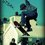# Permutation of Professor's Cube.

There are 98 pieces on the exterior of the cube: 8 corners, 36 edges, and 54 centers (48 movable, 6 fixed).

Any permutation of the corners is possible, including odd permutations, giving 8! possible arrangements. Seven of the corners can be independently rotated, and the orientation of the eighth depends on the other seven, giving 37 combinations.

There are 54 centers. Six of these (the center square of each face) are fixed in position. The rest consist of two sets of 24 centers. Within each set there are four centers of each color. Each set can be arranged in 24! different ways. Assuming that the four centers of each color in each set are indistinguishable, the number of permutations of each set is reduced to 24!/(4!6) arrangements, all of which are possible. The reducing factor comes about because there are 4! ways to arrange the four pieces of a given color. This is raised to the sixth power because there are six colors. The total number of permutations of all movable centers is the product of the permutations of the two sets, 24!2/(4!12).

The 24 outer edges cannot be flipped, since the interior shape of those pieces is asymmetrical. Corresponding outer edges are distinguishable, since the pieces are mirror images of each other. Any permutation of the outer edges is possible, including odd permutations, giving 24! arrangements. The 12 central edges can be flipped. Eleven can be flipped and arranged independently, giving 12!/2 × 211 or 12! × 210 possibilities (an odd permutation of the corners implies an odd permutation of the central edges, and vice versa, thus the division by 2). There are 24! × 12! × 210 possibilities for the inner and outer edges together.

This gives a total number of permutations of

{\frac{8! \times 3^7 \times 12! \times 2^{10} \times 24!^3}{4!^{12}} \approx 2.83 \times 10^{74} The full number is precisely 282 870 942 277 741 856 536 180 333 107 150 328 293 127 731 985 672 134 721 536 000 000 000 000 000 possible permutations[\7] (about 283 duodecillion on the long scale or 283 tresvigintillion on the short scale.Note by Utsav Singhal
6 years, 7 months ago

This discussion board is a place to discuss our Daily Challenges and the math and science related to those challenges. Explanations are more than just a solution — they should explain the steps and thinking strategies that you used to obtain the solution. Comments should further the discussion of math and science.

When posting on Brilliant:

• Use the emojis to react to an explanation, whether you're congratulating a job well done , or just really confused .
• Ask specific questions about the challenge or the steps in somebody's explanation. Well-posed questions can add a lot to the discussion, but posting "I don't understand!" doesn't help anyone.
• Try to contribute something new to the discussion, whether it is an extension, generalization or other idea related to the challenge.

MarkdownAppears as
*italics* or _italics_ italics
**bold** or __bold__ bold
- bulleted- list
• bulleted
• list
1. numbered2. list
1. numbered
2. list
Note: you must add a full line of space before and after lists for them to show up correctly
paragraph 1paragraph 2

paragraph 1

paragraph 2

[example link](https://brilliant.org)example link
> This is a quote
This is a quote
    # I indented these lines
# 4 spaces, and now they show
# up as a code block.

print "hello world"
# I indented these lines
# 4 spaces, and now they show
# up as a code block.

print "hello world"
MathAppears as
Remember to wrap math in $$ ... $$ or $ ... $ to ensure proper formatting.
2 \times 3 $2 \times 3$
2^{34} $2^{34}$
a_{i-1} $a_{i-1}$
\frac{2}{3} $\frac{2}{3}$
\sqrt{2} $\sqrt{2}$
\sum_{i=1}^3 $\sum_{i=1}^3$
\sin \theta $\sin \theta$
\boxed{123} $\boxed{123}$